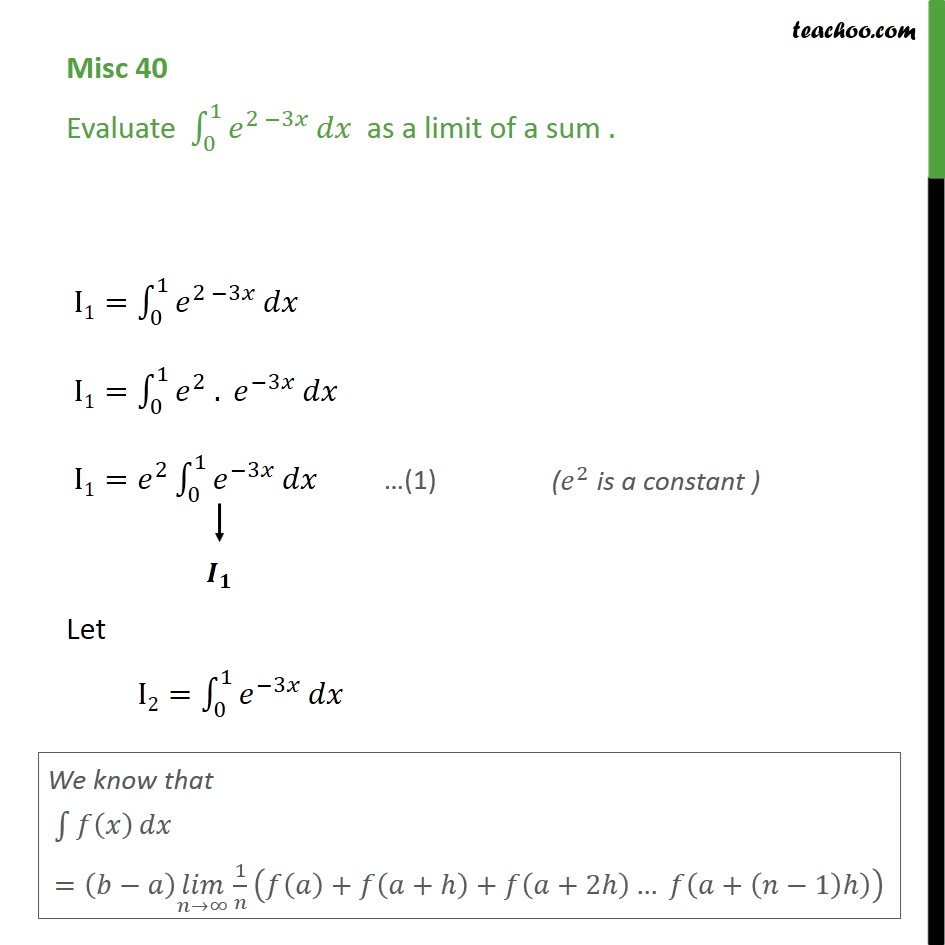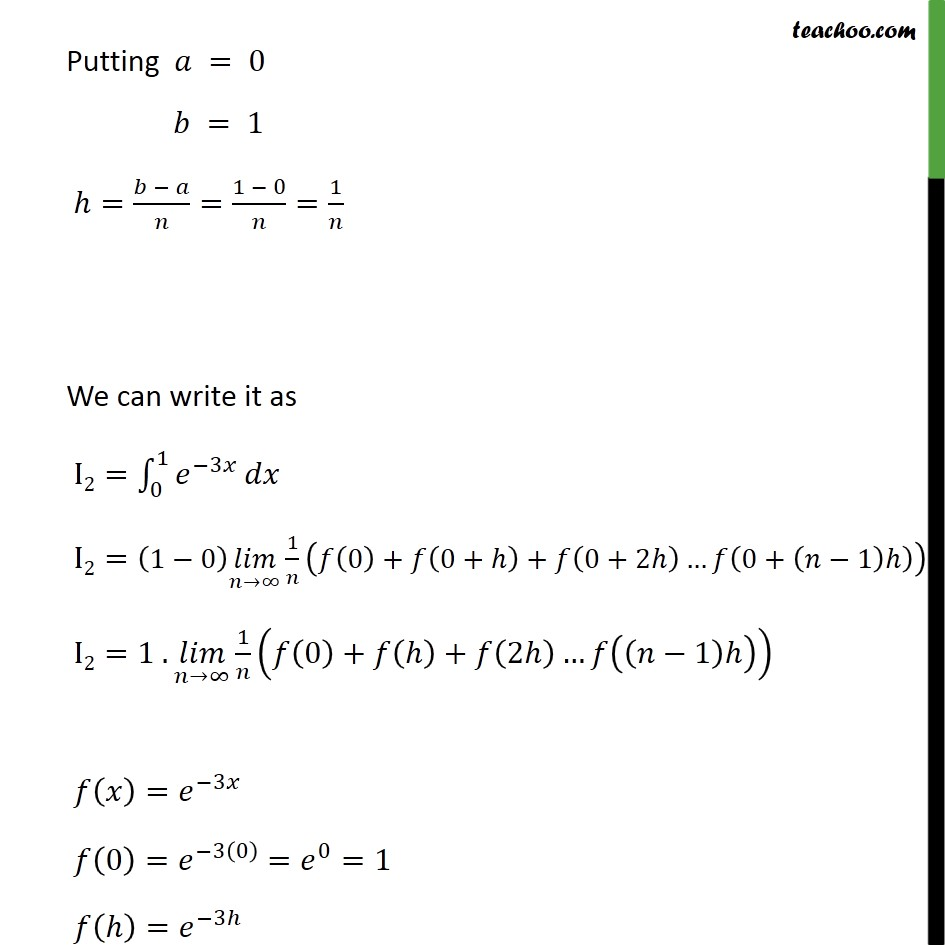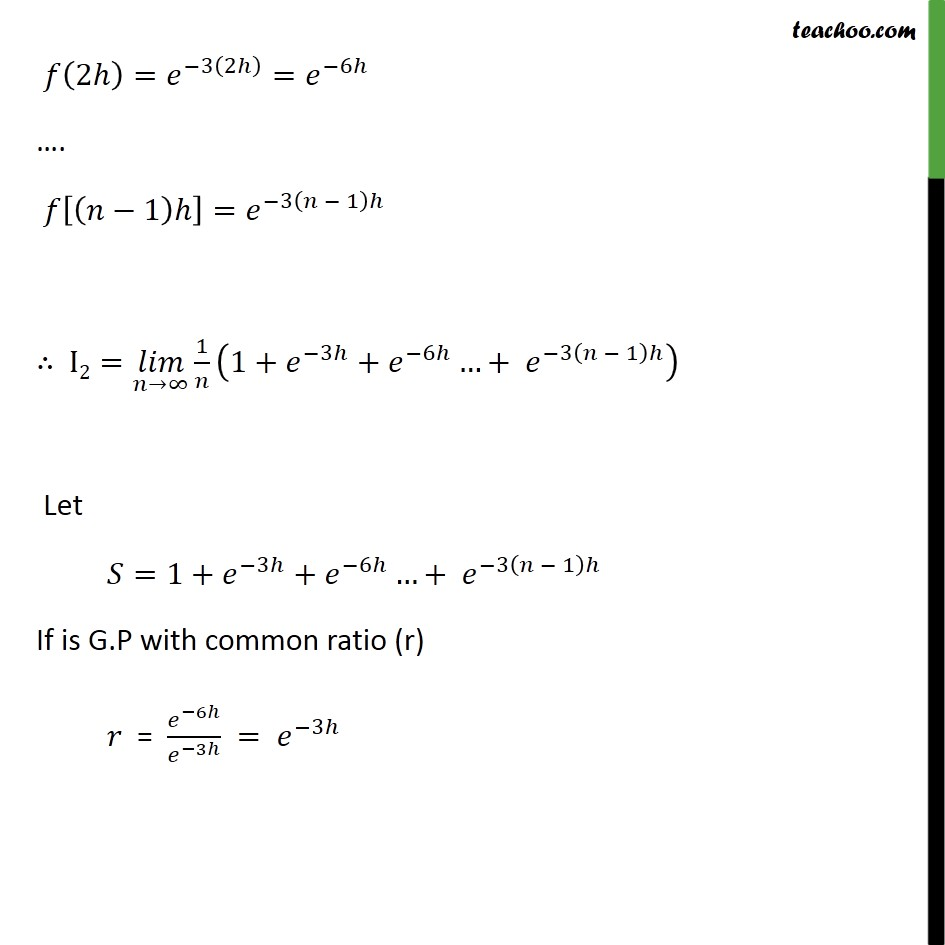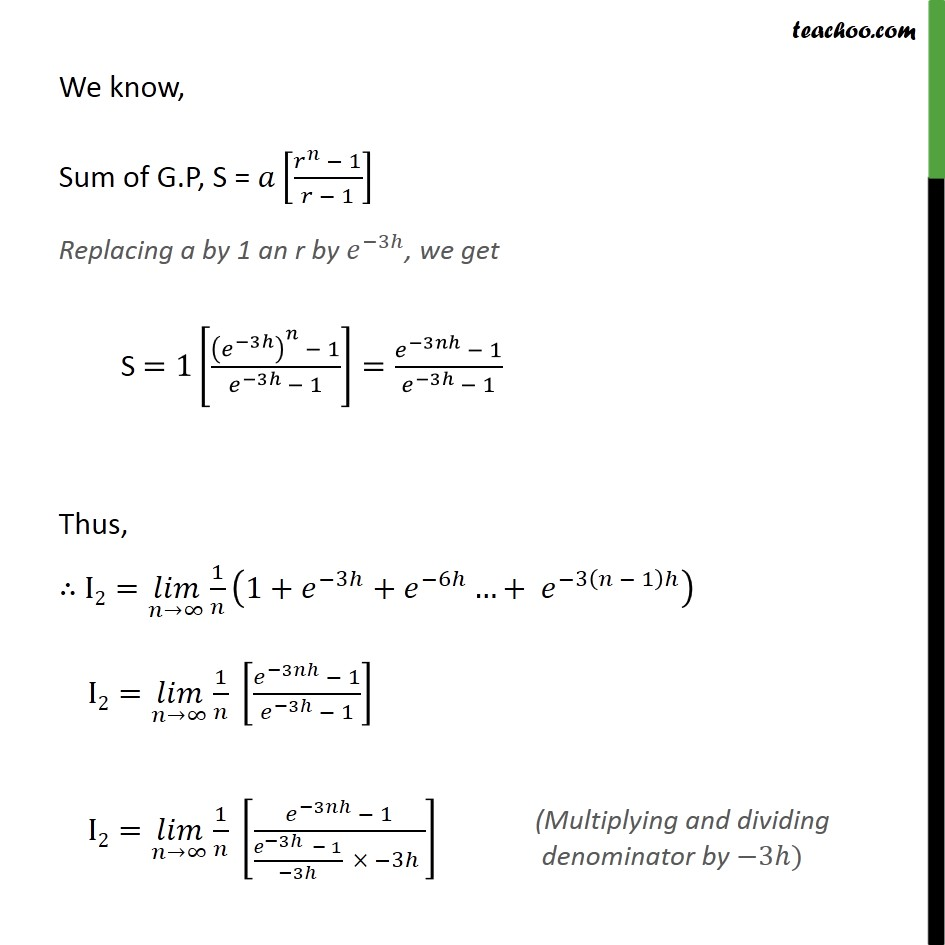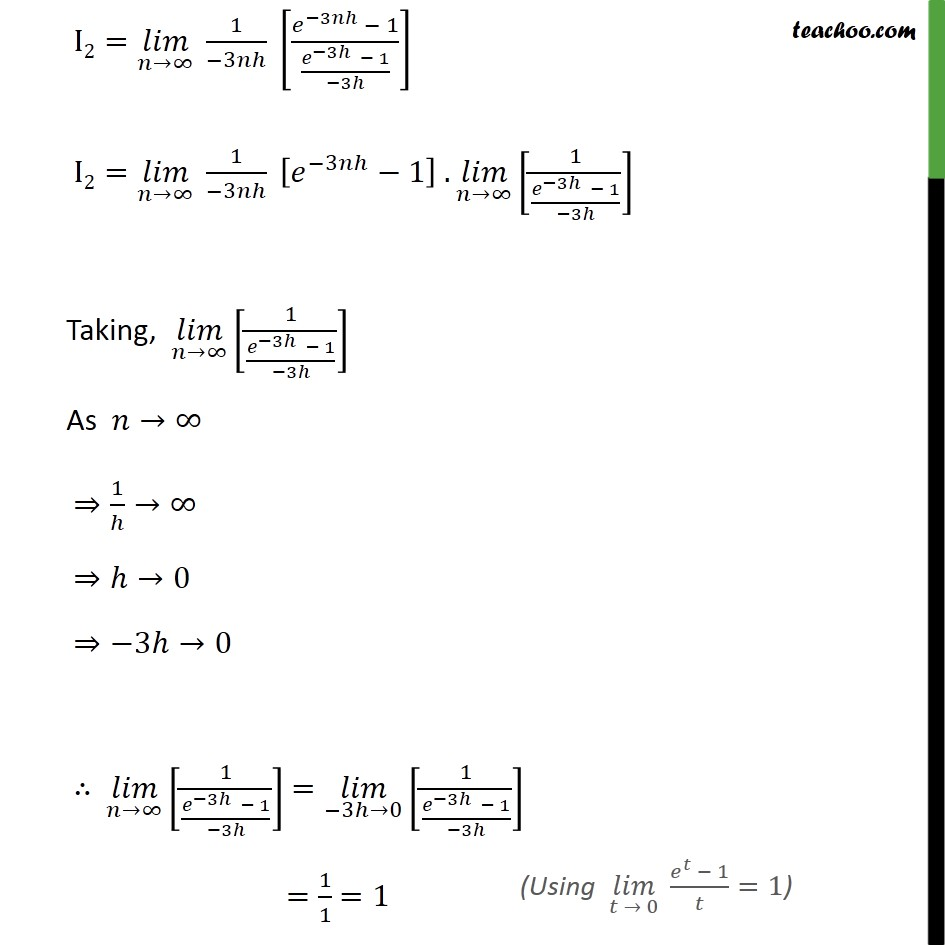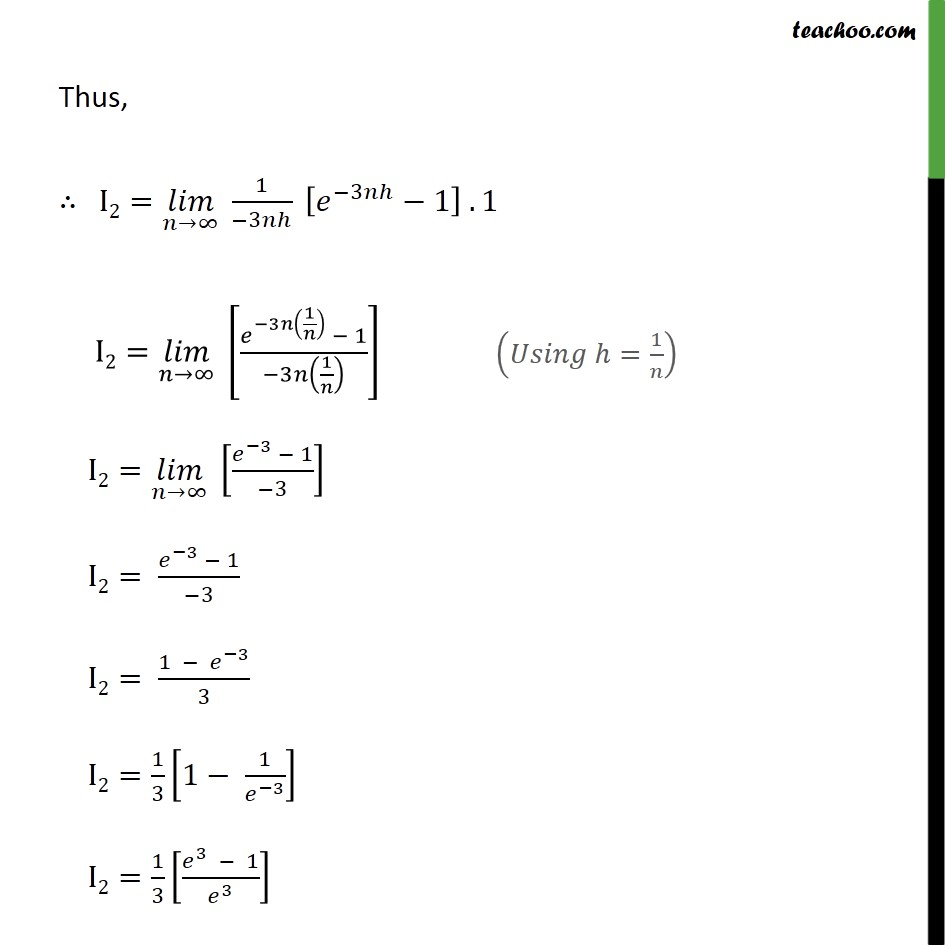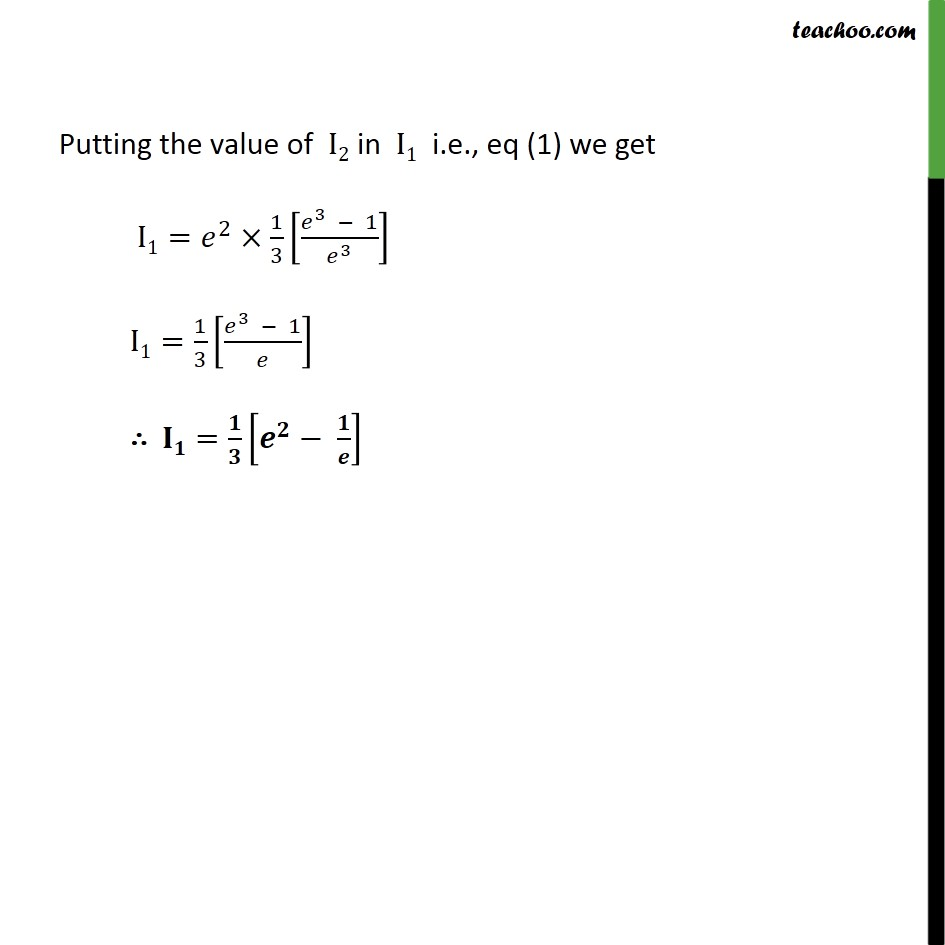1. Chapter 7 Class 12 Integrals
2. Serial order wise
3. Miscellaneous

Transcript

Misc 40 Evaluate 0 1 2 3 as a limit of a sum . I1= 0 1 2 3 I1= 0 1 2 . 3 I1= 2 0 1 3 Let I2= 0 1 3 Putting = 0 = 1 = = 1 0 = 1 We can write it as I2= 0 1 3 I2= 1 0 uc1 1 0 + 0+ + 0+2 0+ 1 I2=1 . uc1 1 0 + + 2 1 = 3 0 = 3 0 = 0 =1 = 3 2 = 3 2 = 6 . 1 = 3 1 I2= uc1 1 1+ 3 + 6 + 3 1 Let =1+ 3 + 6 + 3 1 If is G.P with common ratio (r) = 6 3 = 3 We know, Sum of G.P, S = 1 1 Replacing a by 1 an r by 3 , we get S =1 3 1 3 1 = 3 1 3 1 Thus, I2= uc1 1 1+ 3 + 6 + 3 1 I2= uc1 1 3 1 3 1 I2= uc1 1 3 1 3 1 3 3 I2= uc1 1 3 3 1 3 1 3 I2= uc1 1 3 3 1 . uc1 1 3 1 3 Taking, uc1 1 3 1 3 As 1 0 3 0 uc1 1 3 1 3 = 3 0 1 3 1 3 = 1 1 =1 Thus, I2= uc1 1 3 3 1 . 1 I2= uc1 3 1 1 3 1 I2= uc1 3 1 3 I2= 3 1 3 I2= 1 3 3 I2= 1 3 1 1 3 I2= 1 3 3 1 3 Putting the value of I2 in I1 i.e., eq (1) we get I1= 2 1 3 3 1 3 I1= 1 3 3 1 =

Miscellaneous

Chapter 7 Class 12 Integrals
Serial order wise